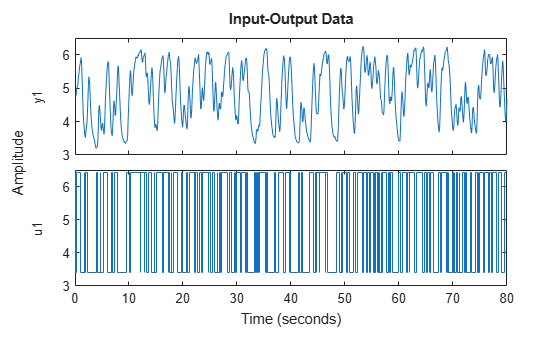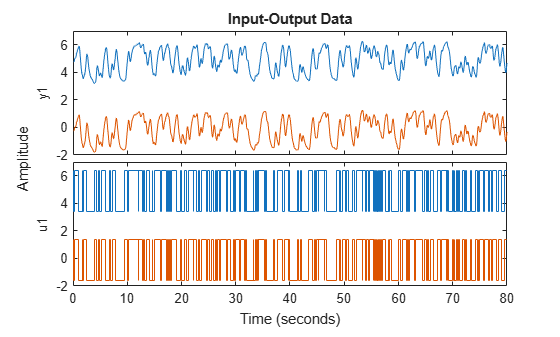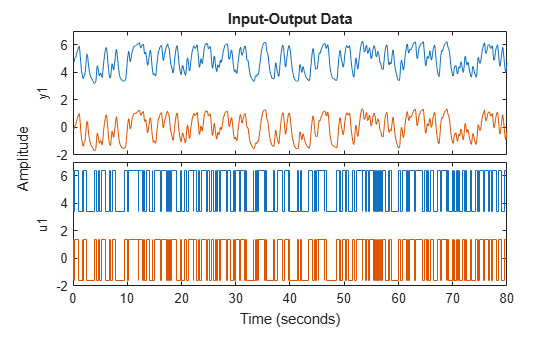# getTrend

Data offset and trend information

## Syntax

```T = getTrend(data) T = getTrend(data,0) T = getTrend(data,1) ```

## Description

`T = getTrend(data)` constructs a `TrendInfo` object to store offset, mean, or linear trend information for detrending or retrending data. You can assign specific offset and slope values to `T`.

`T = getTrend(data,0)` computes the means of input and output signals and stores them as `InputOffset` and `OutputOffset` properties of `T`, respectively.

`T = getTrend(data,1)` computes a best-fit straight line for both input and output signals and stores them as properties of `T`.

## Examples

collapse all

Remove specified offset from input and output signals.

Load SISO data containing vectors `u2` and `y2`.

`load dryer2`

Create a data object with sample time of 0.08 seconds and plot it.

```data = iddata(y2,u2,0.08); plot(data)```The data has a nonzero mean value.

Store the data offset and trend information in a `TrendInfo` object.

`T = getTrend(data);`

Assign offset values to the `TrendInfo` object.

```T.InputOffset = 5; T.OutputOffset = 5;```

Subtract offset from the data.

`data_d = detrend(data,T);`

Plot the detrended data on the same plot.

```hold on plot(data_d)```View the mean value removed from the data.

`get(T)`
```ans = struct with fields: DataName: 'data' InputOffset: 5 OutputOffset: 5 InputSlope: 0 OutputSlope: 0 ```

Compute input-output signal means, store them, and detrend the data.

Load SISO data containing vectors `u2` and `y2`.

`load dryer2`

Create a data object with sample time of 0.08 seconds.

`data = iddata(y2,u2,0.08);`

Compute the mean of the data.

`T = getTrend(data,0);`

Remove the mean from the data.

`data_d = detrend(data,T);`

Plot the original and detrended data on the same plot.

`plot(data,data_d)`